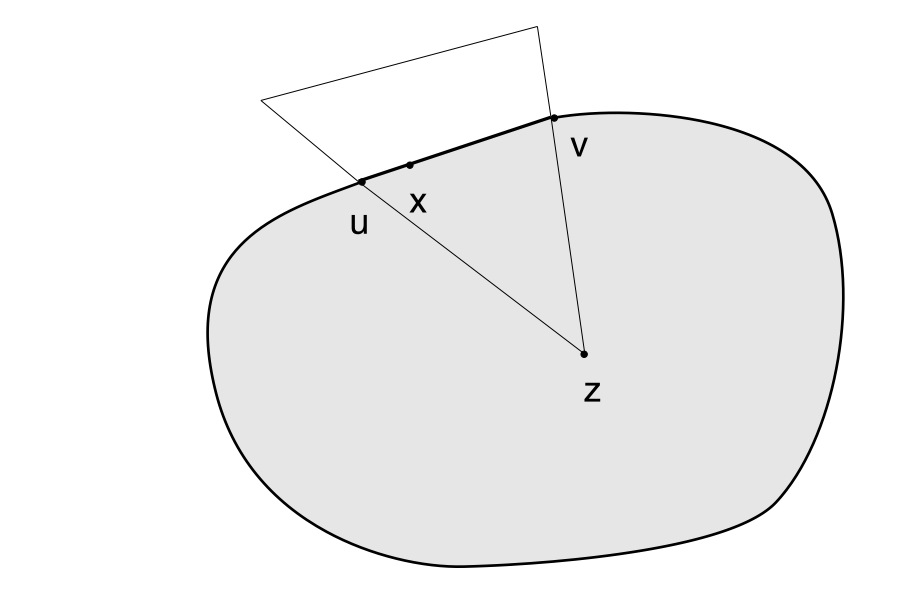# A compact convex set whose extreme points set is not close

Let’s remind that an extreme point $$c$$ of a convex set $$C$$ in a real vector space $$E$$ is a point in $$C$$ which does not lie in any open line segment joining two points of $$C$$.

## The specific case of dimension $$2$$

Proposition: when $$C$$ is closed and its dimension is equal to $$2$$, the set $$\hat{C}$$ of its extreme points is closed.

To prove the proposition, we take a point $$x \notin \hat{C}$$ and demonstrate that $$x$$ belongs to an open set $$O$$ whose intersection with $$\hat{C}$$ is empty. That’s clear when $$x \notin C$$ as $$C$$ is supposed to be closed. If $$x \in C$$, there exist $$u,v \in C$$ distinct such that $$x$$ belongs to the open line segment $$(u,v)$$.

If a point of the segment $$(u,v)$$ is in the interior of $$C$$, $$x$$ itself is in the interior of $$C$$ and therefore belongs to an open disk $$O$$ included in $$C$$. The intersection of $$O$$ with $$\hat{C}$$ is empty as all points of $$O$$ belongs to an open line segment joining two points of $$O \subset C$$.Remains the case where the open line segment $$(u,v)$$ is included in the boundary of $$C$$. In that case consider the figure on the right. We can find an open triangle containing $$x$$, having a vertex $$z$$ in the interior of $$C$$ (as $$C$$ is of dimension $$2$$) and whose intersection with $$\hat{C}$$ is empty. That ends the proof.

## The counterexample in dimension $$3$$ (or higher)In dimension $$3$$ a compact convex $$C$$ can have a set of extreme points $$\hat{C}$$ which is not closed. Take the example of the compact convex consisting of the union of two oblique circular cones like on the left picture. The mid-point $$I$$ of the two vertexes $$A$$ and $$B$$ doesn’t belong to $$\hat{C}$$ while all the other points of the circle $$D$$ are elements of $$\hat{C}$$, therefore $$\hat{C}$$ is not close.